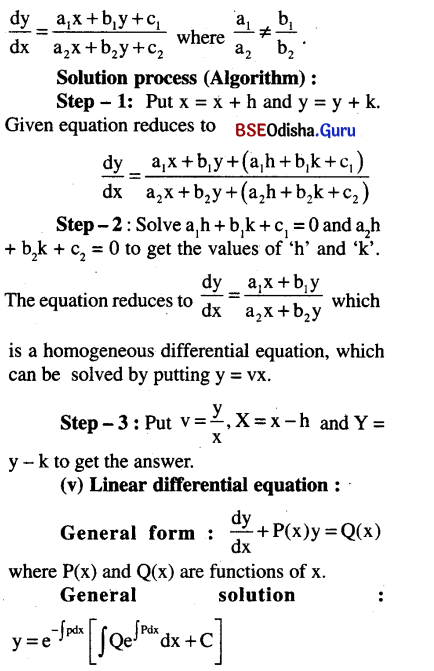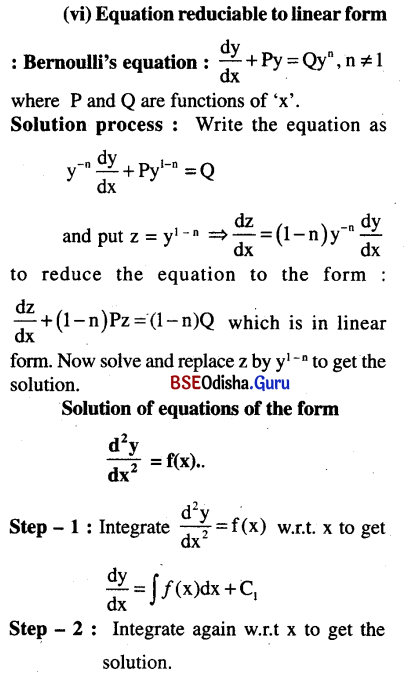# CHSE Odisha Class 12 Math Notes Chapter 11 Differential Equations

Odisha State Board CHSE Odisha Class 12 Math Notes Chapter 11 Differential Equations will enable students to study smartly.

## CHSE Odisha 12th Class Math Notes Chapter 11 Differential Equations

(a) Differential equation:
It is an equation involving independent variables, dependent variables and their derivatives.

(b) Ordinary D.E:
Differential equation that contains derivatives with respect to a single independent variable.

(c) Partial D.E:
Differential equation that contains derivatives with respect to more than one independent variable.

(d) Order of a D.E:
Order of a differential equation is the order of the highest order derivative occurs in the equation.
eg. $$\frac{d^3 y}{d x^3}+\left(\frac{d y}{d x}\right)^5$$+ y = 0 is of order – 3.

(e) Degree of D.E:
Degree of a differential equation is the power of the highest order derivative occurs in the equation after changing to integral powers.
In the above example the degree is ‘1’.(f) Formation of a D.E:
(i) Differential equation for f(x, y, a) = 0 … (1)
can be obtained by eliminating a from (1) and
$$\frac{d}{d x}$$f(x, y, a) = 0 … (2)
(ii) Differential equation for
f(x, y, a, b) = 0 … (1)
can be obtained by eliminating a, b from (1),
$$\frac{d}{d x}$$f(x, y, a, b) = 0 … (2)
and $$\frac{{d}^2}{{dx}^2}$$f(x, y, a, b) = 0 … (3)

(g) Solution of a D.E:
A solution of a differential equation is a function which satisfies the given equation.
General Solution: A solution is a general solution if it contains as many arbitrary constants as the order of the differential equation.
Particular Solution: It is a solution that can be obtained by giving particular values to the arbitrary constants.
Singular Solution: The solutions which can not be obtained from the general solution are singular solution.

(h) First order and first degree differential equation:
Definition:
A first order first degree differential equation takes the form
$$\frac{d y}{d x}$$ = f(x, y)

Standard types and methods of solution:
(i) Variable separable:
If we can express $$\frac{d y}{d x}$$ = f(x, y) in the form N(y) dy = M(x) dx
then we can get a solution by direct integration. The reduced equation is called equation with separable variables.

(ii) Equations reducible to variables separable form:
If the equation is in the form
$$\frac{d y}{d x}$$ = f(ax + by + c) then pur z = ax + by + c to reduce the equation to variable separable form.
The equations of the form $$\frac{d y}{d x}=\frac{a_1 x+b_1 y+c_1}{a_2 x+b_2 y+c_2}$$ where $$\frac{a_1}{a_2}=\frac{b_1}{b_2}$$ can be reduced to variable separable form by putting z = a1x + b2y.

(iii) Homogeneous differential equation:
A differential equation of the form $$\frac{d y}{d x}=\frac{f(x, y)}{g(x, y)}$$ where f(x, y) and g(x, y) are homogeneous function of x, y and of same degree is a homogeneous differential equation.
Solution process:
Put y = vx, $$\frac{d y}{d x}=v+x \frac{d v}{d x}$$ to reduce the equation to variable separable form. Then solve(iv) Equations reduciable to homogeneous form:
Form of the equation:Homogeneous equations:
Let f(x,y) and g(x, y) be homogeneous functions of x and y of same degree.
Then $$\frac{d y}{d x}=\frac{f(x, y)}{g(x, y)}$$ is called a homogeneous differential equation.
For homogeneous differential equation put y = vx and the proceed.